Algebra – Solve for m – Solve the equation – 6 Worksheets

Algebra – Solve for m – Solve the equation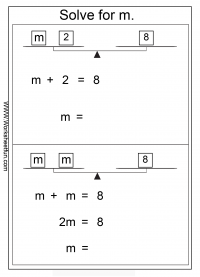Difference between m+2=8 and 2m=8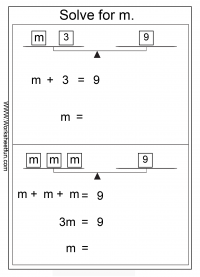Difference between m+3=9 and 3m=9

Difference between m+4=16 and 4m=16

Difference between m+2=6 and 2m=6

Difference between m+3=6 and 3m=6

Difference between m+4=12 and 4m=12

### New Worksheets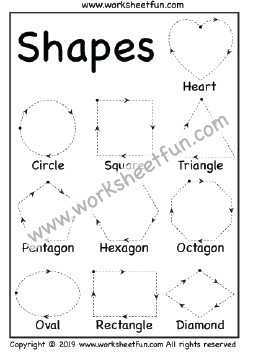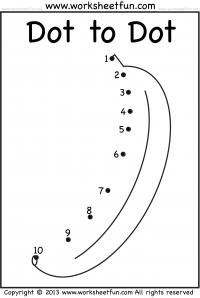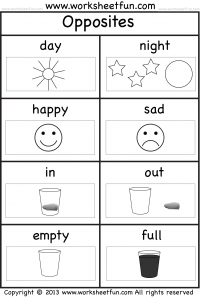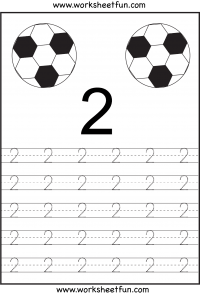### Most Popular Preschool and Kindergarten Worksheets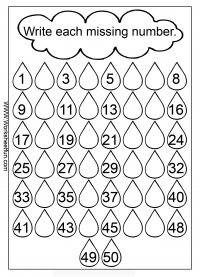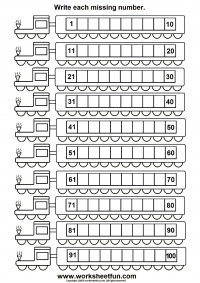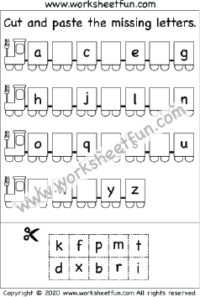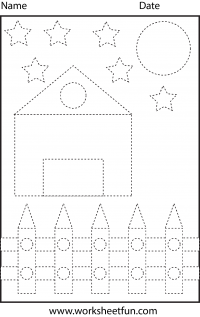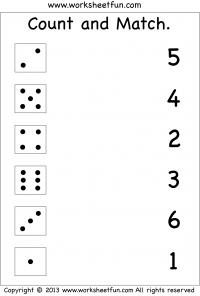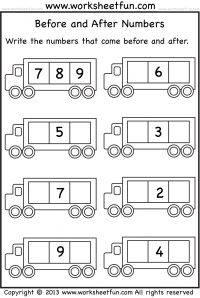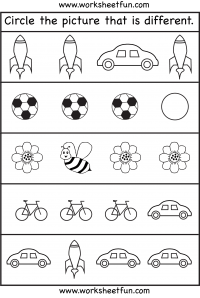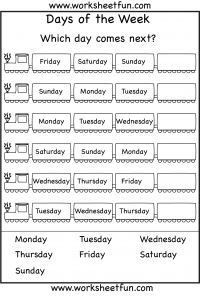#### Kindergarten Worksheets